• 什么是巴纳姆效应呢？就是“每个人都会很容易相信个笼统的、一般性的人格描述特别适合他。即使这种描述十分空洞，仍然认为反映了自己的人格面貌”。在网络上比较流行的星座—性格分析，就是个典型的例子。下面...心理学上有一个效应，叫做“巴纳姆效应”。
什么是巴纳姆效应呢？
就是“每个人都会很容易相信一个笼统的、一般性的人格描述特别适合他。即使这种描述十分空洞，仍然认为反映了自己的人格面貌”。
在网络上比较流行的星座—性格分析，就是一个典型的例子。
下面列举一些典型的巴纳姆语句：
“你祈求受到他人喜爱却对自己吹毛求疵。
虽然人格有些缺陷，大体而言你都有办法弥补。
你拥有可观的未开发潜能，尚未把你的长处完全发挥。
看似强硬、严格自律的外在掩盖着不安与忧虑的内心。
许多时候，你严重的质疑自己是否做了对的事情或正确的决定。
你喜欢一定程度的变动并在受限时感到不满。
你为自己是独立思想者自豪并且不会接受没有充分证据的言论。
你认为对他人过度坦率是不明智的。
有些时候你外向、亲和、充满社会性，有些时候你却内向、谨慎而沉默。”
这些句子，可以得到任何一个人的共鸣。
但这就是问题的关键吗？当然不是！
我们注意，当我们每个人都相信某些特定的描述性句子可以准确地描述自己时，
到底是只是我们“相信”这些句子准确描述了自己，
还是这些句子真的准确描述了自己？
问题的关键的就在这里——这些句子真的准确地描述了我们！
这才是问题的关键！
这就涉及到一个有关人性的重大命题——人性大于性格！
人的共性，我们一定要深刻理解人的共性。
人的共性，远远大于人的差异性。
人的共性，远远大于人的差异性。
我们一定要深刻理解这句话。
我们要知道，人之作为人，人之所以被称为一个物种，
这么多人，这么多各式各样的人，之所以被称为一个物种，
是因为这些家伙在生理机制、心理机制、行为模式、反应模式上面，有着极强极强的共性！
而这些共性，我们将之统称为人性。
人性大于性格！人性远远大于性格！
你必须要深刻地理解这句话，
什么叫深刻地理解这句话，就是你不再认为这个人是内向的，那个人是外向的，
事实是，内向的人也有很多外向的一面，外向的人也有很多内向的一面。
你不再认为这个人是乐观的，那个人是悲观的，
事实是，乐观的人也有悲观的时刻，悲观的人也有乐观的幻想。
人性大于性格！
人性大于性格！
明白了这一点之后，你就会发现，
用“内向”“外向”“好动”“沉默”“乐观”“悲观”“积极”“消极”这些截然相反的词汇来区分人，
是非常低级，非常粗暴，非常没有抓住重点的！
人性大于性格！
好了，本文结束。

展开全文• 在上学期的教学过程中，同学们对“否命题”与“命题的否定”常常混淆不清，在学习四种命题(原命题、逆命题、否命题、逆否命题)时，对于否命题的认识不会有什么疑问，但在学习了或、且、非命题之后，却弄不清楚两者的...


在上学期的教学过程中，同学们对“否命题”与“命题的否定”常常混淆不清，在学习四种命题(原命题、逆命题、否命题、逆否命题)时，对于否命题的认识不会有什么疑问，但在学习了或、且、非命题之后，却弄不清楚两者的区别了，这说明我们在学习过程中对两个概念的理解还不够透彻、不够准确，这里我对“否命题”与“命题的否定”这两个概念做一个详细的阐述。
一、否命题：一个命题是“如果p,那么q”的形式时，它的否命题是既否定条件又否定结论，即“如果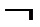p,那么q”。原命题和其否命题的真假关系不确定，可能同真可能同假也可能一真一假。
1．要想写出否命题，需先把原命题改写成“如果(若)……那么(则)……”的形式。
例1：(1)原命题：若三角形中有两边相等，则其对角相等。(真)
否命题：若三角形中有两边不等，则其对角也不相等。(真)
(2)原命题：若两角为对顶角，则此二角相等。(真)
否命题：若两角不是对顶角，则此二角不相等。(假)
(3)原命题：若四边形的四边相等，则为正方形。(假)
否命题：若四边形四边不等，则不是正方形。(真)
(4)原命题：若|x+1|=2，则x=10。(假)
否命题：若|x+1|≠2，则x≠10。(假)
例2：(1)原命题：正方形的四条边相等。
改写为：如果一个四边形为正方形，那么这个四边形的四条边相等。(真)
否命题：如果一个四边形不是正方形，那么这个四边形的四条边不相等。(假)
(2)原命题：负数的绝对值等于它的相反数。
改写为：如果x<0，那么|x|=-x。(真)
否命题：如果x≥0，那么|x|≠-x。(假)
2．如果一个命题没有改写成“如果(若)……那么(则)……”的形式，我们就不能试图去写它的否命题。
例3：(1)2>1；
(2)2+i是复数；
(3)人是生物；
(4)太阳比地球大。
这些命题很难找出条件和结论，无法快捷地写成“如果(若)……那么(则)……”的形式，所以，我们将不再要求写它们的否命题。
其实这些命题不是不能改写成“若……则……”的形式，只是这些命题的条件会用到相应学科的原始概念，是我们不能简单表述的，故不要求同学们在这上面投入无意义的精力。
二、命题的否定：一般地，对于一个命题p全盘否定，就得到一个新命题，记作 “p”，叫做命题p的否定。一个命题与它的否定形式是完全对立的，两者之间有且只有一个成立。命题与其否定的关系，本质上等同于集合与其补集的关系、或者互为对立事件的关系。
对于命题的否定，从以下几种情况进行逐步理解：
1．简单命题的否定：
不含“或”“且”“非”“如果那么”等词语的简单命题，写其否定形式的关键，是否定其中的“谓语部分”，即用来表示判断的词语。下面写出一些常用词语和它的否定词语(前面为原词语，后面为否定词语)：
等于，        不等于；
大于，        不大于(小于或等于)；
小于，        不小于(大于或等于)；
都是，        不都是；
至多有一个，  至少有两个；
至多有n个，   至少有n＋1个；
至少有一个，     一个也没有；
任意的，       某一个；等等。
例4：(1)命题p：2>1(真)；命题p：2≤1(假)；
(2)命题p：2+i是复数(真)；命题p：2+i不是复数(假)；
(3)命题p：1+1≠2(假)；命题p：1+1=2(真)；
(4)命题p：空集是任何集合的真子集(假)；命题p：空集是某些集合的真子集(真)。
2．含有一个逻辑联结词“或(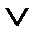)”“且(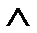)”的命题的否定：
“p且q”的否定是“p或q”； “p或q”的否定是“p且q”。
例5：(1)命题p：2是6的约数且2是4的约数(真)；
命题p：2不是6的约数或2不是4的约数(假)；
(2)命题p：|x-1|=2的实数根是-1或者3；
命题p：|x-1|=2的实数根不是-1而且不是3(假)。
3．全称命题和特称命题命题的否定：
全称量词(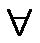)的否定为特称量词(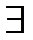)，特称量词的否定为全称量词。写含全称量词或特称量词的命题的否定时，既要否定结论，还要否定相应的量词。
例6：(1)命题p：x∈R，x2>0(假)；
命题p：x∈R，x2≤0(真)；
(2)命题p：有的菱形是正方形(存在)(真)；
命题p：所有的菱形都不是正方形(任意)(假)。
三、(这一条较难理解)条件或结论中含有“或”“ 且”等逻辑联结词的命题的否命题：在否定条件和结论时，注意同时否定联结词。如：
原命题：如果pq，那么st；否命题：如果pq，那么st。(其他情况雷同)
例7：(1)命题p：若x2=1，则x=1或x=-1(真)；
命题p：若x2≠1，则x≠1且x≠-1(真)；
(2)命题p：各内角相等且各边相等的多边形为正多边形，(真)；
命题p：(若)内角不全相等或者各边不全相等的(则)多边形不是正多边形(真)；
(3)命题p：圆内垂直平分弦的直线过圆心且平分该弦所对的弧；(真)
命题p：(若)圆内与弦不垂直或者不平分弦的直线，(则该弦)不过圆心或者不平分线索对的弧。(真)；
(4)命题p：若x=1或者x=2，则x(x-1)=0或者x(x-2)=0(真)；
命题p：若x≠1且x≠2，则x(x-1)≠0且x(x-2)≠0(假)。
四、(这一条非常重要，也是我们最容易弄错的)“如果A，那么B”的否定：这些命题其实可以看做是全称命题“只要A成立，就有B成立”，而全称命题的否定应该是特称命题，故其否定应该是“存在A成立，使B不成立”。这也说明命题的否定并不是简单地只否定结论。
例8：(1)命题p：若x2>4，则x>2(假)；
如果我们认为只需否定结论就能得到p，就会出现这样的情况：
命题p：若x2>4，则x≤2(假)；
p与p竟然都是假命题，这与它们真值相反是矛盾的。
我们换个方法理解命题p：对x2>4，总有x>2(假)，则其否定显然是：
命题p：x2>4，满足x≤2(真)；
(2)命题p：若x2=4，则x=2(假)；
命题p：x满足x2=4，使x≠2(真)；
如果我们用集合的观点理解命题，每个命题实质上对应的都是集合。为了便于表述，不妨把命题A与B对应的集合还用A、B表示，这个时候很容易理解：“若A，则B”等价于“x∈A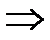x∈B”，那么其否定应为“x∈Ax∉B”。
五、小结：
否命题注重的是形式结构，需先把原命题改写成“如果A，那么B”的形式，再否定条件和结论，得到否命题：“如果A，那么B”；不能改写成“如果……那么……”的命题就不要写否命题。
命题的否定(p)注重本质，必须是相互排斥、对立、互补的，用集合的观点理解，命题的否定就是求补集。写含全称量词或特称量词的命题的否定时，既要否定结论，还要否定相应的量词。“若A，则B”等价于“x∈Ax∈B”，那么其否定应该为“x∈Ax∉B”。
景于行数学手抄报 景于行数学小报 分享提高数学的知识技能
在线小班课 就上景于行 景于行 超级小班 让北京郊区的孩子，在家就能享受海淀教育。港澳台联考
景于行港澳台华侨生联考，传递来自北京的联考教学品质


展开全文• 里面含有的任意和存在量词补充起来，然后同时对量词进行改变； 4.最终一定要确定原命题与命题的否定是否存在你真我假的关系。 以上就是若p则q的命题否定正确做题的逻辑，你会了吗？ 想了解更多精彩内容，快来关注...

大家好，这里是羊爸爸数学课堂，专注分享初高中数学解题技巧与资料！思考背景
​课堂上出现了这么一道题：
命题：若xy=0则x=0或y=0,写出该命题的否定。很多学生和老师看到这道题，立马就想到这样的方法：
若p则q的否命题为若非p则非q；
若p则q命题的否定为若p则非q。
按照这样原则问题的答案为：若xy=0，则x≠0且y≠0
这样写显然是正确答案，也就是说我们前面的方法是对的。

进入正题但是，别急，看下面的这道题：
写出若x²=9则x=3的命题的否定。
先分析这个命题是真还是假，显然此命题为假命题！
那么该命题的否定如何写，答案是：若x²=9则x≠3吗？
明显错了，为什么？
因为x≠3，x=-3也可以！所以此命题也是个假命题！
那我们如何去改写这道命题？
首先我们要了解原命题与否命题以及命题的否定之间的逻辑关系！
原命题：若p则q
否命题：若非p则非q(原命题为真，它可能为真也可能为假，不能确定)
命题的否定：若p则非q(原命题和它的关系一定是你真我假的关系)
所以我们做这样的题一定要有你真我假的思想指导你。
那么具体怎么做？请看：
原命题：对于任意的x，x²=9，则x=3(原命题里面缺乏了一个任意的这个东西)假命题
命题的否定：存在一个x，使得x²=9，则x≠3(这样改写后那么就对了) 真命题
总结1.运用好若p则q命题的否定为若p则非q；
2.用完后看原命题与命题的否定是否存在你真我假的关系(是就对了，不是就要下一步操作)；
3.把里面含有的任意和存在量词补充起来，然后同时对量词进行改变；
4.最终一定要确定原命题与命题的否定是否存在你真我假的关系。
以上就是若p则q的命题否定正确做题的逻辑，你会了吗？
想了解更多精彩内容，快来关注羊爸爸数学课堂

展开全文• 1.4 全称量词与存在量词课时问题提出 1.对于命题p、q，命题p∧q，p∨q，﹁p的含义分别如何？这些命题与p、q的真假关系如何？ p∧q：用联结词“且”命题p和命题q联结起来得到的命题
• ## 离散数学学习笔记【第一篇】

千次阅读 多人点赞 2019-04-03 23:33:15
离散数学学习笔记【第篇】
本文所有内容来自上海科学技术文献出版社《离散数学》第一篇。

目录
第一篇 数理逻辑第一章 命题逻辑1-1 命题及其表示法1-2 联结词1-3 命题公式与翻译1-4 真值表与等价公式1-5 重言式与蕴含式1-6 其他联结词1-7 对偶与范式1-8 推理理论
第二章 谓词逻辑2-1 谓词的概念与表示2-2 命题函数与量词2-3 谓词公式与翻译2-4 变元的约束2-5 谓词演算的等价式与蕴含式2-6 前束范式2-7 谓词演算的推理理论

第一篇 数理逻辑
第一章 命题逻辑
1-1 命题及其表示法
具有确定真值的陈述句叫做命题。 命题有两种类型：不能分解为更简单的陈述语句的称为原子命题；由联结词，标点符号和原子命题复合构成的命题称为复合命题。 命题常用大写字母或带下标的大写字母或数字表示，如

A

A

，

B

i

B_i

，

[

12

]



等,这些符号称为命题标识符。
1-2 联结词

P

P

Q

Q

¬

P

\neg P

P

∧

Q

P \wedge Q

P

∨

Q

P \vee Q

P

→

Q

P \rightarrow Q

P

⇆

Q

P \leftrightarrows Q

T

T

T

T

F

F

T

T

T

T

T

T

T

T

T

T

F

F

F

F

F

F

T

T

F

F

F

F

F

F

T

T

T

T

F

F

T

T

T

T

F

F

F

F

F

F

T

T

F

F

F

F

T

T

T

T

1-3 命题公式与翻译
递归定义了合式公式（

wff

\text{wff}

）
1-4 真值表与等价公式

定义 1 - 4.1 在命题公式中，对于分量指派真值的各种可能组合，就确定了这个命题公式的各种真值情况，把它汇列成表，就是命题公式的真值表。

定义 1 - 4.2 给定两个命题公式

A

A

和

B

B

，设

P

1

,

P

2

,

⋯
&ThinSpace;

,

P

n

P_1,P_2,\cdots,P_n

为所有出现于

A

A

和

B

B

中的原子变元，若给

P

1

,

P

2

,

⋯
&ThinSpace;

,

P

n

P_1,P_2,\cdots,P_n

任一组真值指派，

A

A

和

B

B

的真值都相同，则称

A

A

和

B

B

是等价的或逻辑相等的。记作

A

⇔

B

A\Leftrightarrow B

。

命题定律表达式对合律

¬

¬

P

⇔

P

\neg\neg P \Leftrightarrow P

幂等律

P

∨

P

⇔

P

,

P

∧

P

⇔

P

P \vee P \Leftrightarrow P , P \wedge P \Leftrightarrow P

结合律

(

P

∨

Q

)

∨

R

⇔

P

∨

(

Q

∨

R

)

(

P

∧

Q

)

∧

R

⇔

P

∧

(

Q

∧

R

)

\begin{aligned}(P \vee Q)\vee R \Leftrightarrow P \vee (Q \vee R)\\(P \wedge Q)\wedge R \Leftrightarrow P \wedge (Q \wedge R)\end{aligned}

交换律

P

∨

Q

⇔

Q

∨

P

P

∧

Q

⇔

Q

∧

P

\begin{aligned}P \vee Q \Leftrightarrow Q \vee P\\P \wedge Q \Leftrightarrow Q \wedge P\end{aligned}

分配律

P

∨

(

Q

∧

R

)

⇔

(

P

∨

Q

)

∧

(

P

∨

R

)

P

∧

(

Q

∨

R

)

⇔

(

P

∧

Q

)

∨

(

P

∧

R

)

\begin{aligned}P \vee (Q \wedge R) \Leftrightarrow (P \vee Q)\wedge(P \vee R)\\P \wedge (Q \vee R) \Leftrightarrow (P \wedge Q)\vee(P \wedge R)\end{aligned}

吸收律

P

∨

(

P

∧

Q

)

⇔

P

P

∧

(

P

∨

Q

)

⇔

P

\begin{aligned}P \vee (P \wedge Q) \Leftrightarrow P\\P \wedge (P \vee Q) \Leftrightarrow P\end{aligned}

德·摩根律

¬

(

P

∨

Q

)

⇔

¬

P

∧

¬

Q

¬

(

P

∧

Q

)

⇔

¬

P

∨

¬

Q

\begin{aligned}\neg(P \vee Q) \Leftrightarrow \neg P \wedge \neg Q\\\neg(P \wedge Q) \Leftrightarrow \neg P \vee \neg Q\end{aligned}

同一律

P

∨

F

⇔

P

,

P

∧

T

⇔

P

P \vee \pmb{F} \Leftrightarrow P , P \wedge \pmb{T} \Leftrightarrow P

零律

P

∨

T

⇔

T

,

P

∧

F

⇔

F

P \vee \pmb{T} \Leftrightarrow \pmb{T} , P \wedge \pmb{F} \Leftrightarrow \pmb{F}

否定律

P

∨

¬

P

⇔

T

,

P

∧

¬

P

⇔

F

P \vee \neg P \Leftrightarrow \pmb{T} , P \wedge \neg P \Leftrightarrow \pmb{F}

1-5 重言式与蕴含式

定义 1 - 5.1 给定一命题公式，若无论对分量作怎样的指派，其对应的真值永为

T

\pmb{T}

，则称该命题公式为重言式或永真公式。

定义 1 - 5.2 给定一命题公式，若无论对分量作怎样的指派，其对应的真值永为

F

\pmb{F}

，则称该命题公式为矛盾式或永假公式。

定理 1 - 5.1 任何两个重言式的合取或析取，仍然是一个重言式。

定理 1 - 5.2 一个重言式，对同一分量都用任何合式公式置换，其结果仍为一重言式。

定理 1 - 5.3 设

A

A

和

B

B

为两个命题公式，

A

⇔

B

A \Leftrightarrow B

当且仅当

A

⇆

B

A \leftrightarrows B

为一个重言式。

定义 1 - 5.3 当且仅当

P

→

Q

P \rightarrow Q

是一个重言式时，我们称“

P

P

蕴含

Q

Q

”，并记作

P

⇒

Q

P \Rightarrow Q

。

定理 1 - 5.4 设

P

P

和

Q

Q

为任意两个命题公式，

P

⇔

Q

P \Leftrightarrow Q

的充分必要条件是

P

⇒

Q

P \Rightarrow Q

且

Q

⇒

P

Q \Rightarrow P

。

1-6 其他联结词

P

P

Q

Q

P

∨

‾

Q

P \overline{\vee} Q

P

→

c

Q

P \overset{c}{\rightarrow} Q

P

↑

Q

P \uparrow Q

P

↓

Q

P \downarrow Q

T

T

T

T

F

F

F

F

F

F

F

F

T

T

F

F

T

T

T

T

T

T

F

F

F

F

T

T

T

T

F

F

T

T

F

F

F

F

F

F

F

F

F

F

T

T

T

T

P

∨

‾

Q

⇔

(

P

∧

¬

Q

)

∨

(

¬

P

∧

Q

)

P

→

c

Q

⇔

P

∧

¬

Q

P

↑

Q

⇔

¬

P

∨

¬

Q

P

↓

Q

⇔

¬

P

∧

¬

Q

P \overline{\vee} Q \Leftrightarrow (P \wedge \neg Q) \vee (\neg P \wedge Q)\\ P \overset{c}{\rightarrow} Q \Leftrightarrow P \wedge \neg Q\\ P \uparrow Q \Leftrightarrow \neg P \vee \neg Q\\ P \downarrow Q \Leftrightarrow \neg P \wedge \neg Q

1-7 对偶与范式

定义 1 - 7.1 在给定的命题公式中，将联结词

∨

\vee

换成

∧

\wedge

，将

∧

\wedge

换成

∨

\vee

，若有特殊变元

T

\pmb{T}

和

F

\pmb{F}

亦相互取代，所得公式

A

∗

A^*

称为

A

A

的对偶式。

定理 1 - 7.1 设

A

A

和

A

∗

A^*

是对偶式，

P

1

,

P

2

,

⋯
&ThinSpace;

,

P

n

P_1,P_2,\cdots,P_n

是出现在

A

A

和

A

∗

A^*

中的原子变元，则

¬

A

(

P

1

,

P

2

,

⋯
&ThinSpace;

,

P

n

)

⇔

A

∗

(

¬

P

1

,

¬

P

2

,

⋯
&ThinSpace;

,

¬

P

n

)

A

(

¬

P

1

,

¬

P

2

,

⋯
&ThinSpace;

,

¬

P

n

)

⇔

¬

A

∗

(

P

1

,

P

2

,

⋯
&ThinSpace;

,

P

n

)

\begin{aligned}\neg A(P_1,P_2,\cdots,P_n)\Leftrightarrow A^*(\neg P_1,\neg P_2,\cdots,\neg P_n)\\A(\neg P_1,\neg P_2,\cdots,\neg P_n)\Leftrightarrow \neg A^*(P_1,P_2,\cdots,P_n)\end{aligned}

定理 1 - 7.2 设

P

1

,

P

2

,

⋯
&ThinSpace;

,

P

n

P_1,P_2,\cdots,P_n

是出现在公式

A

A

和

B

B

中的所有原子变元，如果

A

⇔

B

A\Leftrightarrow B

，则

A

∗

⇔

B

∗

A^*\Leftrightarrow B^*

。

定义 1 - 7.2 一个命题公式称为合取范式，当且仅当它具有型式：

A

1

∧

A

2

∧

⋯

∧

A

n

,

(

n

⩾

1

)

A_1 \wedge A_2 \wedge \cdots \wedge A_n , (n \geqslant 1)

其中

A

1

,

A

2

,

⋯
&ThinSpace;

,

A

n

A_1,A_2,\cdots,A_n

都是由命题变元或其否定所组成的析取式。

定义 1 - 7.3 一个命题公式称为析取范式，当且仅当它具有型式：

A

1

∨

A

2

∨

⋯

∨

A

n

,

(

n

⩾

1

)

A_1 \vee A_2 \vee \cdots \vee A_n , (n \geqslant 1)

其中

A

1

,

A

2

,

⋯
&ThinSpace;

,

A

n

A_1,A_2,\cdots,A_n

都是由命题变元或其否定所组成的合取式。

定义 1 - 7.4

n

n

个命题变元的合取式，称作布尔合取或小项，其中每个变元与它的否定不能同时存在，但两者必须出现且仅出现一次。

定义 1 - 7.5 对于给定的命题公式，如果有一个等价公式，它仅由小项的析取所组成，则该等价式称作原式的主析取范式。

定理 1 - 7.3 在真值表中，一个公式的真值为

T

\pmb{T}

的指派所对应的小项的析取，即为此公式的主析取范式。

定义 1 - 7.6

n

n

个命题变元的析取式，称作布尔析取或大项，其中每个变元与它的否定不能同时存在，但两者必须出现且仅出现一次。

定义 1 - 7.7 对于给定的命题公式，如果有一个等价公式，它仅由大项的合取所组成，则该等价式称作原式的主合取范式。

定理 1 - 7.4 在真值表中，一个公式的真值为

F

\pmb{F}

的指派所对应的大项的合取，即为此公式的主合取范式。

例题 化

(

P

∧

Q

)

∨

(

¬

P

∧

R

)

(P \wedge Q) \vee (\neg P \wedge R)

为主合取范式和主析取范式。 解：

(

P

∧

Q

)

∨

(

¬

P

∧

R

)

⇔

(

P

∨

¬

P

)

∧

(

P

∨

R

)

∧

(

Q

∨

¬

P

)

∧

(

Q

∨

R

)

⇔

(

P

∨

R

∨

(

Q

∧

¬

Q

)

)

∧

(

¬

P

∨

Q

∨

(

R

∧

¬

R

)

)

∧

(

(

P

∧

¬

P

)

∨

Q

∨

R

)

⇔

(

P

∨

Q

∨

R

)

∧

(

P

∨

¬

Q

∨

R

)

∧

(

¬

P

∨

Q

∨

R

)

∧

(

¬

P

∨

Q

∨

¬

R

)

⇔

∏

2

,

3

,

5

,

7

⇔

∑

0

,

1

,

4

,

6

\begin{aligned}&amp;(P \wedge Q) \vee (\neg P \wedge R)\\\Leftrightarrow&amp;(P \vee \neg P) \wedge (P \vee R)\wedge(Q \vee \neg P)\wedge(Q \vee R)\\\Leftrightarrow&amp;(P \vee R \vee (Q \wedge \neg Q))\wedge(\neg P \vee Q \vee (R \wedge \neg R))\wedge((P \wedge \neg P)\vee Q \vee R)\\\Leftrightarrow&amp;(P \vee Q \vee R)\wedge(P \vee \neg Q \vee R)\wedge(\neg P \vee Q \vee R)\wedge(\neg P \vee Q \vee \neg R)\\\Leftrightarrow&amp;\prod\nolimits_{2,3,5,7}\\\Leftrightarrow&amp;\sum\nolimits_{0,1,4,6}\end{aligned}

1-8 推理理论

定义 1 - 8.1 设

A

A

和

C

C

是两个命题公式，当且仅当

A

→

C

A\rightarrow C

为一重言式，即

A

⇒

C

A \Rightarrow C

，称

C

C

是

A

A

的有效结论。或

C

C

可由

A

A

逻辑地推出。 设

H

1

,

H

2

,

⋯
&ThinSpace;

,

H

n

,

C

H_1,H_2,\cdots,H_n,C

是命题公式，当且仅当

H

1

∧

H

2

∧

⋯

∧

H

n

⇒

C

H_1 \wedge H_2 \wedge \cdots \wedge H_n \Rightarrow C

，称

C

C

是一组前提

H

1

,

H

2

,

⋯
&ThinSpace;

,

H

n

H_1,H_2,\cdots,H_n

的有效结论。

判别有效结论的过程就是论证过程，基本方法为真值表法、直接证法和间接证法。
（1）真值表法
（2）直接证法

P

P

规则：前提在推导过程中的任何时候都可以引入使用。

T

T

规则：在推导中，如果有一个或多个公式、重言蕴含着公式

S

S

，则公式

S

S

可以引入推导之中。
例题 证明

(

W

∨

R

)

→

V

,

V

→

C

∨

S

,

S

→

U

,

¬

C

∧

¬

U

⇒

¬

W

(W \vee R)\rightarrow V , V\rightarrow C \vee S , S \rightarrow U , \neg C \wedge \neg U \Rightarrow \neg W

。 证明：

(

1

)

¬

C

∧

¬

U

P

(

2

)

¬

U

T

(

1

)

I

(

3

)

S

→

U

P

(

4

)

¬

S

T

(

2

)

,

(

3

)

I

(

5

)

¬

C

T

(

1

)

I

(

6

)

¬

C

∧

¬

S

T

(

4

)

,

(

5

)

I

(

7

)

¬

(

C

∨

S

)

T

(

6

)

E

(

8

)

(

W

∨

R

)

→

V

P

(

9

)

V

→

(

C

∨

S

)

P

(

10

)

(

W

∨

R

)

→

(

C

∨

S

)

T

(

8

)

,

(

9

)

I

(

11

)

¬

(

W

∨

R

)

T

(

7

)

,

(

10

)

I

(

12

)

¬

W

∧

¬

R

T

(

11

)

E

(

13

)

¬

W

T

(

12

)

I

\begin{aligned}&amp;(1)\neg C \wedge \neg U &amp;P\\ &amp;(2)\neg U &amp;T(1)I\\ &amp;(3)S\rightarrow U &amp;P\\ &amp;(4)\neg S &amp;T(2),(3)I\\ &amp;(5)\neg C &amp;T(1)I\\ &amp;(6)\neg C \wedge \neg S &amp;T(4),(5)I\\ &amp;(7)\neg(C \vee S) &amp;T(6)E\\ &amp;(8)(W \vee R) \rightarrow V &amp;P\\ &amp;(9)V \rightarrow (C \vee S) &amp;P\\ &amp;(10)(W \vee R) \rightarrow (C \vee S) &amp;T(8),(9)I\\ &amp;(11)\neg(W \vee R) &amp;T(7),(10)I\\ &amp;(12)\neg W \wedge \neg R &amp;T(11)E\\ &amp;(13)\neg W &amp;T(12)I \end{aligned}

（3）间接证法

定义 1 - 8.2 假设公式

H

1

,

H

2

,

⋯
&ThinSpace;

,

H

m

H_1,H_2,\cdots,H_m

中的命题变元为

P

1

,

P

2

,

⋯
&ThinSpace;

,

P

n

P_1,P_2,\cdots,P_n

，对于

P

1

,

P

2

,

⋯
&ThinSpace;

,

P

n

P_1,P_2,\cdots,P_n

的一些真值指派，如果能使

H

1

∧

H

2

∧

⋯

∧

H

m

H_1 \wedge H_2 \wedge \cdots \wedge H_m

的真值为

T

\pmb{T}

，则称公式

H

1

,

H

2

,

⋯
&ThinSpace;

,

H

m

H_1,H_2,\cdots,H_m

是相容的。如果对于

P

1

,

P

2

,

⋯
&ThinSpace;

,

P

n

P_1,P_2,\cdots,P_n

的每一组真值指派使得

H

1

∧

H

2

∧

⋯

∧

H

m

H_1 \wedge H_2 \wedge \cdots \wedge H_m

的真值均为

F

\pmb{F}

，则称公式

H

1

,

H

2

,

⋯
&ThinSpace;

,

H

m

H_1,H_2,\cdots,H_m

是不相容的。

要证

H

1

∧

H

2

∧

⋯

∧

H

m

⇒

C

H_1 \wedge H_2 \wedge \cdots \wedge H_m\Rightarrow C

，即证

H

1

,

H

2

,

⋯
&ThinSpace;

,

H

m

H_1,H_2,\cdots,H_m

与

¬

C

\neg C

是不相容的 (将

¬

C

\neg C

作为

P

（

附

加

条

件

）

P（附加条件）

)。
要证

S

⇒

(

R

→

C

)

S\Rightarrow (R \rightarrow C)

，即证

(

S

∧

R

)

⇒

C

(S \wedge R)\Rightarrow C

（

C

P

CP

规则）。
第二章 谓词逻辑
2-1 谓词的概念与表示
我们用大写字母表示谓词，用小写字母表示客体名称，如

A

(

b

)

A(b)

、

B

(

a

,

b

)

B(a,b)

、

L

(

a

,

b

,

c

)

L(a,b,c)

等。 单独一个谓词不是完整的命题，我们把谓词字母后填以客体所得的式子称为谓词填式，如果

A

A

为

n

n

元谓词，

a

1

,

a

2

,

⋯
&ThinSpace;

,

a

n

a_1,a_2,\cdots,a_n

是客体的名称，则

A

(

a

1

,

a

2

,

⋯
&ThinSpace;

,

a

n

)

A(a_1,a_2,\cdots,a_n)

就可成为命题。
2-2 命题函数与量词

定义 2 - 2.1 由一个谓词和一些客体变元组成的表达式，称为简单命题函数。

命题函数确定为命题，与客体变元的论述范围有关。在命题函数中，客体变元的论述范围称作个体域。个体域可以是有限的，也可以是无限的，把各种个体域综合在一起作为论述范围的域称全总个体域。
引入两种量词，一个用符号

(

∀

x

)

(\forall x)

或

(

x

)

(x)

表示，代表“对所有的

x

x

”，称为全称量词；另一个用符号

(

∃

x

)

(\exist x)

表示，表示“存在一些

x

x

”，称为存在量词。全称量词和存在量词同称为量词。
为了方便，我们将所有命题函数的个体域全部统一，一律使用全总个体域。
2-3 谓词公式与翻译

定义 2 - 3.1 谓词演算的合式公式，可由下述各条组成： （1）原子谓词公式是合式公式。 （2）若

A

A

是合式公式，则

¬

A

\neg A

是一个合式公式。 （3）若

A

A

和

B

B

都是合式公式，则

(

A

∧

B

)

(A\wedge B)

、

(

A

∨

B

)

(A \vee B)

、

(

A

→

B

)

(A \rightarrow B)

和

(

A

⇆

B

)

(A \leftrightarrows B)

是合式公式。 （4）如果

A

A

是合式公式，

x

x

是

A

A

中出现的任何变元，则

(

∀

x

)

A

(\forall x)A

和

(

∃

x

)

A

(\exist x)A

都是合式公式。 （5）只有经过有限次地应用规则(1),(2),(3),(4)得到的公式是合式公式。

2-4 变元的约束
给定

α

\alpha

为一个谓词公式，其中有一部分公式形式为

(

∀

x

)

P

(

x

)

(\forall x)P(x)

或

(

∃

x

)

P

(

x

)

(\exist x)P(x)

。这里

∀

\forall

和

∃

\exist

后面所跟的

x

x

叫做量词的指导变元或作用变元，

P

(

x

)

P(x)

叫做相应量词的作用域或辖域。在作用域中

x

x

的一切出现，称为

x

x

在

α

\alpha

中的约束出现，

x

x

也称为被相应量词中的指导变元所约束。在

α

\alpha

中除去约束变元以外所出现的变元称作自由变元。自由变元可看作是公式中的参数。
设

P

(

x

1

,

x

2

,

⋯
&ThinSpace;

,

x

n

)

P(x_1,x_2,\cdots,x_n)

是

n

n

元谓词，它有

n

n

个相互独立的自由变元，若对其中

k

k

个变元进行约束，则成为

n

−

k

n-k

元谓词。例如，

(

∀

x

)

P

(

x

,

y

,

z

)

(\forall x)P(x,y,z)

是二元谓词，

(

∃

y

)

(

∀

x

)

P

(

x

,

y

,

z

)

(\exist y)(\forall x)P(x,y,z)

是一元谓词。
一个公式的约束变元所使用的名称符号是无关紧要的，

(

∃

x

)

P

(

x

)

(\exist x)P(x)

和

(

∃

y

)

P

(

y

)

(\exist y)P(y)

意义相同。因此，我们可以对公式

α

\alpha

中的约束变元更改名称符号，这种遵守一定规则的更改，称为约束变元的换名。其规则为： （1）对于约束变元可以换名，其更改的变元名称范围是量词中的指导变元，以及该量词作用域中所出现的该变元，在公式的其余部分不变。 （2）换名时一定要更改为作用域中没有出现的变元名称。 举例来说，公式

(

∀

x

)

(

P

(

x

)

→

R

(

x

,

y

)

)

∧

Q

(

x

,

y

)

(\forall x)(P(x)\rightarrow R(x,y))\wedge Q(x,y)

可换名为

(

∀

z

)

(

P

(

z

)

→

R

(

z

,

y

)

)

∧

Q

(

x

,

y

)

(\forall z)(P(z)\rightarrow R(z,y)) \wedge Q(x,y)

对于公式中的自由变元，也允许更改，这种更改叫做代入。自由变元的代入，也需遵守一定的规则，这个规则叫做自由变元的代入规则，说明如下： （1）对于谓词公式中的自由变元，可以作代入，代入时需对公式中出现该自由变元的每一处进行。 （2）用以代入的变元与原公式中的所有变元的名称不能相同。
2-5 谓词演算的等价式与蕴含式

定义 2 - 5.1 给定任何两个谓词公式

wff

A

\text{wff } A

和

wff

B

\text{wff } B

，设它们有共同的个体域

E

E

，若对

A

A

和

B

B

的任一组变元进行赋值，所得命题的真值相同，则称谓词公式

A

A

和

B

B

在

E

E

上是等价的，并记作

A

⇔

B

A \Leftrightarrow B

。

定义 2 - 5.2 给定任意谓词公式

wff

A

\text{wff } A

，其个体域为

E

E

，对于

A

A

的所有赋值，

wff

A

\text{wff } A

都为真，则称

wff

A

\text{wff } A

在

E

E

上是有效的（或永真的）。

定义 2 - 5.3 一个谓词公式

wff

A

\text{wff } A

，如果在所有赋值下都为假，则称该

wff

A

\text{wff } A

为不可满足的。

定义 2 - 5.3 一个谓词公式

wff

A

\text{wff } A

，如果至少在一种赋值下为真，则称该

wff

A

\text{wff } A

为可满足的。

（1）命题公式的推广
命题演算中的等价公式表和蕴含式表都可推广到谓词演算中使用。例如

(

∀

x

)

(

P

(

x

)

→

Q

(

x

)

)

⇔

(

∀

x

)

(

¬

P

(

x

)

∨

Q

(

x

)

)

(

∀

x

)

P

(

x

)

∨

(

∃

y

)

R

(

x

,

y

)

⇔

¬

(

¬

(

∀

x

)

P

(

x

)

∧

¬

(

∃

y

)

R

(

x

,

y

)

)

(\forall x)(P(x)\rightarrow Q(x))\Leftrightarrow(\forall x)(\neg P(x)\vee Q(x)) \\ (\forall x)P(x)\vee(\exist y)R(x,y) \Leftrightarrow \neg(\neg(\forall x)P(x)\wedge \neg (\exist y)R(x,y))

（2）量词与联结词

¬

\neg

之间的关系

¬

(

∀

x

)

P

(

x

)

⇔

(

∃

x

)

¬

P

(

x

)

¬

(

∃

x

)

P

(

x

)

⇔

(

∀

x

)

¬

P

(

x

)

\neg(\forall x)P(x)\Leftrightarrow (\exist x)\neg P(x) \\ \neg(\exist x)P(x)\Leftrightarrow(\forall x)\neg P(x)

约定出现在量词之前的否定不是否定量词而是否定被量化了的整个命题。
（3）量词作用域的扩张与收缩
量词的作用域中如果含有合取项或析取项，则当其中一项为命题时，可将该命题移至量词作用域之外，比如

(

∀

x

)

(

A

(

x

)

∨

B

)

⇔

(

(

∀

x

)

A

(

x

)

∨

B

)

(\forall x)(A(x)\vee B) \Leftrightarrow ((\forall x)A(x)\vee B)

因为在

B

B

中不出现约束变元

x

x

。类似的式子还有

(

(

∀

x

)

A

(

x

)

→

B

)

⇔

(

∃

x

)

(

A

(

x

)

→

B

)

(

(

∃

x

)

A

(

x

)

→

B

)

⇔

(

∀

x

)

(

A

(

x

)

→

B

)

(

B

→

(

∀

x

)

A

(

x

)

)

⇔

(

∀

x

)

(

B

→

A

(

x

)

)

(

B

→

(

∃

x

)

A

(

x

)

)

⇔

(

∃

x

)

(

B

→

A

(

x

)

)

((\forall x)A(x)\rightarrow B)\Leftrightarrow(\exist x)(A(x)\rightarrow B) \\ ((\exist x)A(x)\rightarrow B)\Leftrightarrow(\forall x)(A(x)\rightarrow B) \\ (B\rightarrow(\forall x)A(x))\Leftrightarrow(\forall x)(B\rightarrow A(x)) \\ (B\rightarrow(\exist x)A(x))\Leftrightarrow(\exist x)(B\rightarrow A(x))

（4）量词与命题联结词之间的一些等价式

(

∀

x

)

(

A

(

x

)

∧

B

(

x

)

)

⇔

(

∀

x

)

A

(

x

)

∧

(

∀

x

)

B

(

x

)

(

∃

x

)

(

A

(

x

)

∨

B

(

x

)

)

⇔

(

∃

x

)

A

(

x

)

∨

(

∃

x

)

B

(

x

)

(\forall x)(A(x)\wedge B(x))\Leftrightarrow(\forall x)A(x)\wedge(\forall x)B(x) \\ (\exist x)(A(x)\vee B(x))\Leftrightarrow(\exist x)A(x)\vee(\exist x)B(x)

（5）量词与命题联结词之间的一些蕴含式

(

∀

x

)

A

(

x

)

∨

(

∀

x

)

B

(

x

)

⇒

(

∀

x

)

(

A

(

x

)

∨

B

(

x

)

)

(

∃

x

)

(

A

(

x

)

∧

B

(

x

)

)

⇒

(

∃

x

)

A

(

x

)

∧

(

∃

x

)

B

(

x

)

(

∀

x

)

(

A

(

x

)

→

B

(

x

)

)

⇒

(

∀

x

)

A

(

x

)

→

(

∀

x

)

B

(

x

)

(

∀

x

)

(

A

(

x

)

⇆

B

(

x

)

)

⇒

(

∀

x

)

A

(

x

)

⇆

(

∀

x

)

B

(

x

)

(\forall x)A(x)\vee(\forall x)B(x)\Rightarrow(\forall x)(A(x)\vee B(x)) \\ (\exist x)(A(x)\wedge B(x))\Rightarrow(\exist x)A(x)\wedge(\exist x)B(x) \\ (\forall x)(A(x)\rightarrow B(x))\Rightarrow(\forall x)A(x)\rightarrow(\forall x)B(x) \\ (\forall x)(A(x)\leftrightarrows B(x))\Rightarrow(\forall x)A(x)\leftrightarrows(\forall x)B(x)

（6）多个量词的使用

(

∀

x

)

(

∀

y

)

A

(

x

,

y

)

⇔

(

∀

y

)

(

∀

x

)

A

(

x

,

y

)

(

∃

x

)

(

∃

y

)

A

(

x

,

y

)

⇔

(

∃

y

)

(

∃

x

)

A

(

x

,

y

)

(

∀

x

)

(

∀

y

)

A

(

x

,

y

)

⇒

(

∃

y

)

(

∀

x

)

A

(

x

,

y

)

⇒

(

∀

x

)

(

∃

y

)

A

(

x

,

y

)

⇒

(

∃

x

)

(

∃

y

)

A

(

x

,

y

)

(\forall x)(\forall y)A(x,y) \Leftrightarrow (\forall y)(\forall x)A(x,y) \\ (\exist x)(\exist y)A(x,y) \Leftrightarrow (\exist y)(\exist x)A(x,y) \\ \begin{aligned}(\forall x)(\forall y)A(x,y)&amp;\Rightarrow (\exist y)(\forall x)A(x,y) \\ &amp;\Rightarrow(\forall x)(\exist y)A(x,y)\\&amp;\Rightarrow(\exist x)(\exist y)A(x,y)\end{aligned}

2-6 前束范式

定义 2 - 6.1 一个公式，如果量词均在全式的开头，它们的作用域，延申到整个公式的末尾，则该公式叫做前束范式。

定理 2 - 6.1 任意一个谓词公式，均和一个前束范式等价。

例题 化公式

(

∀

x

)

(

∀

y

)

(

(

∃

z

)

(

P

(

x

,

z

)

∧

P

(

y

,

z

)

)

→

(

∃

u

)

Q

(

x

,

y

,

u

)

)

(\forall x)(\forall y)((\exist z)(P(x,z)\wedge P(y,z))\rightarrow(\exist u)Q(x,y,u))

为前束范式。 解：否定深入

原

式

⇔

(

∀

x

)

(

∀

y

)

(

¬

(

∃

z

)

(

P

(

x

,

z

)

∧

P

(

y

,

z

)

)

∨

(

∃

u

)

Q

(

x

,

y

,

u

)

)

⇔

(

∀

x

)

(

∀

y

)

(

∀

z

)

(

∃

u

)

(

¬

P

(

x

,

z

)

∨

¬

P

(

y

,

z

)

∨

Q

(

x

,

y

,

u

)

)

\begin{aligned}原式&amp;\Leftrightarrow(\forall x)(\forall y)(\neg(\exist z)(P(x,z)\wedge P(y,z))\vee(\exist u)Q(x,y,u))\\&amp;\Leftrightarrow(\forall x)(\forall y)(\forall z)(\exist u)(\neg P(x,z)\vee\neg P(y,z)\vee Q(x,y,u))\end{aligned}

定义 2 - 6.2 （定义了什么叫前束合取范式）

定理 2 - 6.2 每一个

wff

A

\text{wff }A

都可转化为与其等价的前束合取范式。

例题 化

wff

D

\text{wff }D

：

(

∀

x

)

[

(

∀

y

)

P

(

x

)

∨

(

∀

z

)

q

(

z

,

y

)

→

¬

(

∀

y

)

R

(

x

,

y

)

]

(\forall x)\left[(\forall y)P(x)\vee(\forall z)q(z,y)\rightarrow\neg(\forall y)R(x,y)\right]

为前束合取范式。 解：取消多余量词→换名→消去条件联结词→否定深入→量词推到最左边

D

⇔

(

∀

x

)

[

P

(

x

)

∨

(

∀

z

)

q

(

z

,

y

)

→

¬

(

∀

y

)

R

(

x

,

y

)

]

⇔

(

∀

x

)

[

P

(

x

)

∨

(

∀

z

)

q

(

z

,

y

)

→

¬

(

∀

w

)

R

(

x

,

w

)

]

⇔

(

∀

x

)

{

¬

[

P

(

x

)

∨

(

∀

z

)

q

(

z

,

y

)

]

∨

¬

(

∀

w

)

R

(

x

,

w

)

}

⇔

(

∀

x

)

[

¬

P

(

x

)

∧

(

∃

z

)

¬

q

(

z

,

y

)

∨

(

∃

w

)

¬

R

(

x

,

w

)

]

⇔

(

∀

x

)

(

∃

z

)

(

∃

w

)

[

(

¬

P

(

x

)

∨

¬

R

(

x

,

w

)

)

∧

(

¬

q

(

z

,

y

)

∨

¬

R

(

x

,

w

)

)

]

\begin{aligned}D&amp;\Leftrightarrow(\forall x)\left[P(x)\vee(\forall z)q(z,y)\rightarrow\neg(\forall y)R(x,y)\right]\\&amp;\Leftrightarrow(\forall x)\left[P(x)\vee(\forall z)q(z,y)\rightarrow\neg(\forall w)R(x,w)\right]\\&amp;\Leftrightarrow(\forall x)\left\{\neg [P(x)\vee(\forall z)q(z,y)]\vee\neg(\forall w)R(x,w)\right\}\\&amp;\Leftrightarrow(\forall x)[\neg P(x)\wedge(\exist z)\neg q(z,y)\vee(\exist w)\neg R(x,w)]\\&amp;\Leftrightarrow(\forall x)(\exist z)(\exist w)[(\neg P(x)\vee\neg R(x,w))\wedge(\neg q(z,y)\vee\neg R(x,w))]\end{aligned}

定义 2 - 6.3 （定义了什么叫前束析取范式）

定理 2 - 6.3 每一个

wff

A

\text{wff }A

都可转化为与其等价的前束析取范式。

2-7 谓词演算的推理理论
（1）全称指定规则，即

U

S

US

规则。

(

∀

x

)

P

(

x

)

∴

P

(

c

)

（2）全程推广规则，即

U

G

UG

规则。

P

(

x

)

∴

(

∀

x

)

P

(

x

)

（3）存在指定规则，即

E

S

ES

规则。

(

∃

x

)

P

(

x

)

∴

P

(

c

)

（4）存在推广规则，即

E

G

EG

规则。

P

(

c

)

∴

(

∃

x

)

P

(

x

)

例题 证明

(

∀

x

)

(

C

(

x

)

→

W

(

x

)

∧

R

(

x

)

)

∧

(

∃

x

)

(

C

(

x

)

∧

Q

(

x

)

)

⇒

(

∃

x

)

(

Q

(

x

)

∧

R

(

x

)

)

\begin{aligned}&amp;(\forall x)(C(x)\rightarrow W(x)\wedge R(x))\wedge(\exist x)(C(x)\wedge Q(x)) \\ &amp;\Rightarrow(\exist x)(Q(x)\wedge R(x))\end{aligned}

证明：

(

1

)

(

∀

x

)

(

C

(

x

)

→

W

(

x

)

∧

R

(

x

)

)

P

(

2

)

(

∃

x

)

(

C

(

x

)

∧

Q

(

x

)

)

P

(

3

)

C

(

a

)

∧

Q

(

a

)

E

S

(

2

)

(

4

)

C

(

a

)

→

W

(

a

)

∧

R

(

a

)

U

S

(

1

)

(

5

)

C

(

a

)

T

(

3

)

I

(

6

)

W

(

a

)

∧

R

(

a

)

T

(

4

)

,

(

5

)

I

(

7

)

Q

(

a

)

T

(

3

)

I

(

8

)

R

(

a

)

T

(

6

)

(

9

)

Q

(

a

)

∧

R

(

a

)

T

(

7

)

,

(

8

)

I

(

10

)

(

∃

x

)

(

Q

(

x

)

∧

R

(

x

)

)

E

G

\begin{aligned} (1)&amp;(\forall x)(C(x)\rightarrow W(x)\wedge R(x))&amp;P\\ (2)&amp;(\exist x)(C(x)\wedge Q(x))&amp;P\\ (3)&amp;C(a)\wedge Q(a)&amp;ES(2)\\ (4)&amp;C(a) \rightarrow W(a)\wedge R(a)&amp;US(1)\\ (5)&amp;C(a)&amp;T(3)I\\ (6)&amp;W(a)\wedge R(a)&amp;T(4),(5)I\\ (7)&amp;Q(a)&amp;T(3)I\\ (8)&amp;R(a)&amp;T(6)\\ (9)&amp;Q(a)\wedge R(a)&amp;T(7),(8)I\\ (10)&amp;(\exist x)(Q(x)\wedge R(x))&amp;EG \end{aligned}


展开全文离散数学
• 自我感觉量词是正则表达式里最不容易理解的地方，所以特别为它做了个总结。 为了容易理解，会简单地结合正则表达式引擎的工作方式来讲。正则表达式引擎分为文本导向型（Text-directed Engines）...、没有量词时正...正则表达式
• SQL全称量词使用存在量词替代 【例3.62】查询选修了全部课程的学生姓名 SELECT Sname FROM Student WHERE NOT EXISTS ( SELECT * FROM Course WHERE NOT EXISTS ( SELECT * FROM SC WHERE Sno = Student.Sno ...
• 它们的形态虽然不同于{m,n}，功能却是相同的（也可以它们理解为"量词简记法"），具体说明见表2-2。 表2-2 常用量词 常用量词 {m,n}等价形式 说明 * {0,} 可能出现，也...
• ## SQL全称量词

千次阅读 2019-04-22 20:34:01
存在量词 EXISTS谓词： 带有EXISTS谓词的子查询不返回任何数据，只产生逻辑真值“true”或逻辑假值“false”。 若内层查询结果非空，则外层的WHERE子句返回真值； 若内层查询结果为空，则外层的WHERE子句返回假值。...
• 翻译说明 greedy: 贪婪型, 最大匹配方式;... 这3种量词, 是修饰量词量词, 可以理解为正则格式重复的匹配类型。 量词 量词(Quantifier)用来指定某部分正则所重复的次数。为了方便，本文分别介绍 Pattern Ajava 正则表达式
• 数据库之什么时候使用全称量词和存在量词 每次做元组演算的题目的时候都不知道到底什么时候用存在量词什么时候用全称量词，可能你的各个元祖关系写对了，可错在符号，就很难受???? 我就查了一些别人写的博客，借鉴...
• 我们知道，在正则表达式中，可以使用[0-9]或\d来匹配单个数字字符，但是，如果需要验证个更复杂的字符串呢，比如大陆地区的邮政编码。不过邮政编码并没有特别规定，只有由6个数字组成的字符串而已， 如246512，...
• ，也就是匹配优先量词加上个?，忽略优先也叫做我们平时说的惰性匹配 忽略优先量词也是DFA和NFA的分水岭，这个东西是NFA里特有的，DFA里没有的。 忽略优先量词与匹配优先量词不同的地方在于，匹配优先量词会首先...
• 这个量词，引擎会它之前的token匹配次或者零次，换句话说问号之前的token是可选的。 星号的意思是匹配0次或者多次它之前的token，而加号的意思说匹配1次或者多次。例如&lt;[A-Za-z][A-Za-z0-9]*&gt;可以...正则 加号 贪婪模式
• . 谓词逻辑相关概念 1. 个体词 ( 1 ) 个体词 2. 谓词 ( 1 ) 谓词 3. 量词 ( 1 ) 全称量词 ( 2 ) 存在量词 二. 命题符号化 技巧 1. 两个基本公式 ( 1 ) 有性质 F 的个体 都有性质 G ( 2 ) 存在既有性质 ...谓词逻辑
• 3、 量词、 二、 一阶谓词逻辑公式、 三、 两个基本公式、 1、 公式、 2、 公式二、 四、 命题符号化技巧、 1、 命题符号化方法、 2、 谓词逻辑组合、 3、 当且仅当谓词逻辑、 五、 命题符号化示例、谓词逻辑 命题符号化
• MySQL查询之全称量词现在来假设个场景，共有三张表。分表如下： 学生表（student） 课程表（course） 学生_课程对应表（sc） 这时候，有个需求就是查询选修了所有课程的学生的名字。 那么，我们看看都有...
•javascript
• 本文属于「离散数学」系列文章之。这系列着重于离散数学的学习和应用。由于内容随时可能发生更新变动，欢迎关注和收藏离散数学系列文章汇总目录一文以作备忘。此外，在本系列学习文章中，为了透彻理解数学知识，...人工智能 机器学习
• 最后2个其实很好理解，无论量词贴近A(x)还是在括号外，对x的约束没变，因此相等: 7)B→∀xA(x)⇔∀x(B→A(x)) 8)B→∃xA(x)⇔∃x(B→A(x)) 证明只需要用到蕴涵等价式，把量词移到外面即可。 最后，我想可能有人有...
• 它们的形态虽然不同于{m,n}，功能却是相同的（也可以它们理解为“量词简记法”），具体说明见表2-2。   表2-2 常用量词 常用量词 {m,n}等价形式 说明 * {0,} 可能...正则表达式
• 量词的表达前言1.描述“一群”2.描述“丝”、“缕”或“层”3.描述“一阵”4.神奇的piece5.a pair of6. 最常用量词 前言 量词是用以表示事物单位的词，在中文和英文中都非常重要，对人理解相应的具象事物有很...经验分享
• 章 正则表达式字符匹配攻略 正则表达式是匹配模式，要么匹配字符，要么匹配位置。请记住这句话。 然而关于正则如何匹配字符的学习，大部分人都觉得这块比较杂乱。 毕竟元字符太多了，看起来没有系统性，不好...
• 从表2-4可以看到，匹配优先量词与忽略优先量词逐一对应，只是在对应的匹配优先量词之后添加 ? ，两者限定的元素能出现的次数也一样，遇到不能匹配的情况同样需要回溯； 唯一的区别在于，忽略优先量词会优先选择"忽略...
• 所以，先把量词的一些小发现放到这里。 总的来说，量词是辅助我们识别基础维度变化的。 另个重要发现是，就算是基础维度，也是可以进行再次抽象的。 下面的表主要反映了量词与基础维度变化描述的关系 基础...
• ## 量词

2007-09-02 12:32:00
量词（quantifier）允许你指定要匹配的出现次数。为了方便起见，Pattern API规范的三个部分...＋的功能完全一样，因为它们都匹配“X，次或者完全没有”。在本节快结束时将解释它们实现的微妙区别。表13-3 量 词regex search string input 正则表达式
• 正则表达式中有三个量词：贪婪、惰性、支配，记录一下我对贪婪和惰性的理解 1 贪婪：下子打算匹配整个字符串，如果发现不匹配，就去掉最后个字符，进行匹配，以此类推。一旦匹配成功之后，并不是马上返回，而是...正则表达式
• 个人   한 사람 两位老师   두 분의 선생님 三名学生   세 명의 학생 ◆匹  ： 말을 세는 양사 匹马   한 필의 말 ◆头  : 소나 덩치가 큰 동물을 세는 양사 ......